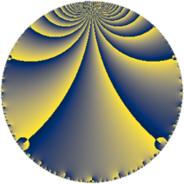# Properties

 Label 74.4.fLevel $74$ Weight $4$ Character orbit 74.f Rep. character $\chi_{74}(7,\cdot)$ Character field $\Q(\zeta_{9})$ Dimension $54$ Newform subspaces $2$ Sturm bound $38$ Trace bound $1$

# Related objects

## Defining parameters

 Level: $$N$$ $$=$$ $$74 = 2 \cdot 37$$ Weight: $$k$$ $$=$$ $$4$$ Character orbit: $$[\chi]$$ $$=$$ 74.f (of order $$9$$ and degree $$6$$) Character conductor: $$\operatorname{cond}(\chi)$$ $$=$$ $$37$$ Character field: $$\Q(\zeta_{9})$$ Newform subspaces: $$2$$ Sturm bound: $$38$$ Trace bound: $$1$$ Distinguishing $$T_p$$: $$3$$

## Dimensions

The following table gives the dimensions of various subspaces of $$M_{4}(74, [\chi])$$.

Total New Old
Modular forms 186 54 132
Cusp forms 162 54 108
Eisenstein series 24 0 24

## Trace form

 $$54 q + 12 q^{3} - 15 q^{5} - 150 q^{7} - 24 q^{8} - 96 q^{9} + O(q^{10})$$ $$54 q + 12 q^{3} - 15 q^{5} - 150 q^{7} - 24 q^{8} - 96 q^{9} + 60 q^{10} + 66 q^{11} + 48 q^{12} - 204 q^{13} + 12 q^{14} - 210 q^{15} - 51 q^{17} - 222 q^{19} - 60 q^{20} + 738 q^{21} + 504 q^{23} - 525 q^{25} + 414 q^{26} + 870 q^{27} + 192 q^{28} + 120 q^{29} + 144 q^{30} + 744 q^{31} - 528 q^{33} - 1014 q^{34} - 750 q^{35} + 1512 q^{36} - 2130 q^{37} - 1776 q^{38} - 978 q^{39} - 48 q^{40} - 789 q^{41} + 840 q^{42} + 288 q^{43} + 168 q^{44} + 1560 q^{45} + 708 q^{46} + 444 q^{47} + 288 q^{48} + 2130 q^{49} - 972 q^{50} + 552 q^{52} + 1038 q^{53} + 720 q^{54} + 2520 q^{55} - 3930 q^{57} - 738 q^{58} + 3096 q^{59} + 2262 q^{61} - 1584 q^{62} - 750 q^{63} - 1728 q^{64} + 249 q^{65} + 450 q^{67} + 768 q^{68} - 10860 q^{69} - 1512 q^{70} + 3426 q^{71} - 3534 q^{73} - 3030 q^{74} - 3456 q^{75} - 888 q^{76} + 1464 q^{77} - 60 q^{78} + 1590 q^{79} - 768 q^{80} - 2040 q^{81} + 1584 q^{82} - 1296 q^{83} + 1008 q^{84} + 2745 q^{85} + 456 q^{86} + 600 q^{87} - 864 q^{88} + 3732 q^{89} + 5358 q^{90} + 9036 q^{91} + 2736 q^{92} - 1164 q^{93} + 6288 q^{94} + 11274 q^{95} + 6804 q^{97} + 360 q^{98} + 420 q^{99} + O(q^{100})$$

## Decomposition of $$S_{4}^{\mathrm{new}}(74, [\chi])$$ into newform subspaces

Label Dim. $$A$$ Field CM Traces $q$-expansion
$$a_2$$ $$a_3$$ $$a_5$$ $$a_7$$
74.4.f.a $$24$$ $$4.366$$ None $$0$$ $$6$$ $$-9$$ $$-75$$
74.4.f.b $$30$$ $$4.366$$ None $$0$$ $$6$$ $$-6$$ $$-75$$

## Decomposition of $$S_{4}^{\mathrm{old}}(74, [\chi])$$ into lower level spaces

$$S_{4}^{\mathrm{old}}(74, [\chi]) \cong$$ $$S_{4}^{\mathrm{new}}(37, [\chi])$$$$^{\oplus 2}$$